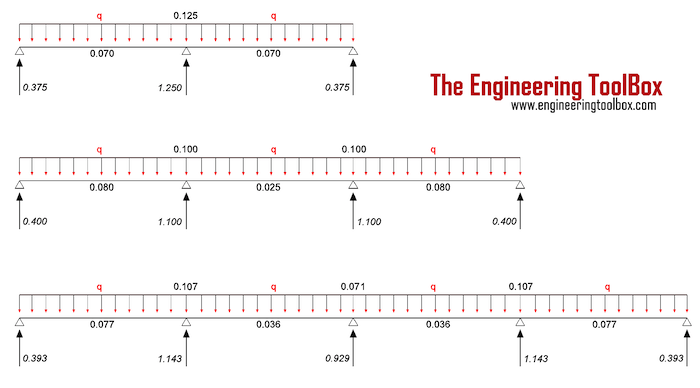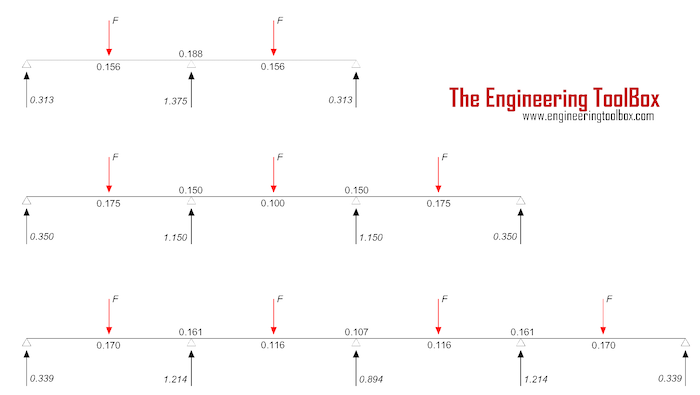Engineering ToolBox - Resources, Tools and Basic Information for Engineering and Design of Technical Applications!

# Continuous Beams - Moment and Reaction Support Forces

## Moments and reaction support forces with distributed or point loads.

### Continuous Beam with Distributed LoadFor a continuous beam with 3, 4 or 5 supports and distributed load the reaction support forces can be calculated as

R = cr q L                     (1)

where

R = reaction support force (N, lbf)

cr = reaction support force coefficient from the figure above

q = distributed load (N/m, lbf/ft)

L = span length (m, ft)

The moments can be calculated as

M = cm q L2                     (2)

where

M = beam moment (Nm, lbf ft)

cm = moment coefficient from the figure above

#### Example - Continuous Beam with Distributed Load

The reaction forces in the end supports for a continuous beam with 3 supports and distributed load 1000 N/m can be calculated as

Rend = (0.375) (1000 N/m)

= 375 N

= 0.38 kN

The reaction force in the center support can be calculated as

Rcenter = (1.250) (1000 N/m)

= 1250 N

= 1.25 kN

The beam moments at the middle of spans with span length 1m can be calculated as

Mend = (0.070) (1000 N/m) (1 m)2

= 70 Nm

The beam moment at the center support can be calculated as

Mcenter = (0.125) (1000 N/m) (1 m)2

= 125 Nm

### Continuous Beam with Point LoadsFor a continuous beam with 3, 4 or 5 supports and point loads the reaction support forces can be calculated as

R = cr F                      (3)

where

cr = reaction support force coefficient from the figure above

F = point load (N, lbf)

The moments can be calculated as

M = cm F L                     (4)

where

cm = moment coefficient from the figure above

#### Example - Continuous Beam with Point Loads

The reaction forces in the end supports for a continuous beam with 3 supports and 2 point loads 1000 N can be calculated as

Rend = (0.313) (1000 N)

= 313 N

= 0.31 kN

The reaction force in the center support can be calculated as

Rcenter = (1.375) (1000 N)

= 1375 N

= 1.4 kN

The beam moments at point loads with span length 1m can be calculated as

Mend = (0.156) (1000 N) (1 m)

= 156 Nm

The beam moment at the center support can be calculated as

Mcenter = (0.188) (1000 N) (1 m)

= 188 Nm

## Related Topics

• ### Beams and Columns

Deflection and stress, moment of inertia, section modulus and technical information of beams and columns.

## Related Documents

• ### Beam Loads - Support Force Calculator

Calculate beam load and supporting forces.

• ### British Universal Columns and Beams

Properties of British Universal Steel Columns and Beams.
• ### Cantilever Beams - Moments and Deflections

Maximum reaction forces, deflections and moments - single and uniform loads.
• ### Drawbridge - Force and Moment vs. Elevation

Calculate the acting forces and moments when elevating drawbridges or beams.
• ### HE-A Steel Beams

Properties of HE-A profiled steel beams.
• ### HE-B Steel Beams

Properties of HE-B profiled steel beams.
• ### Three-Hinged Arches - Continuous and Point Loads

Support reactions and bending moments.
• ### Wood Beams - Strength of Material

Bending and compression strength of wood species used in beams.

## Search

Search is the most efficient way to navigate the Engineering ToolBox.

## Engineering ToolBox - SketchUp Extension - Online 3D modeling!

Add standard and customized parametric components - like flange beams, lumbers, piping, stairs and more - to your Sketchup model with the Engineering ToolBox - SketchUp Extension - enabled for use with older versions of the amazing SketchUp Make and the newer "up to date" SketchUp Pro . Add the Engineering ToolBox extension to your SketchUp Make/Pro from the Extension Warehouse !

We don't collect information from our users. More about

## Citation

• The Engineering ToolBox (2017). Continuous Beams - Moment and Reaction Support Forces . [online] Available at: https://www.engineeringtoolbox.com/continuous-beam-moment-reaction-support-forces-distributed-point-loads-d_1988.html [Accessed Day Month Year].

Modify the access date according your visit.

12.8.9